# Path Loss in Remote Keyless Entry Systems

### Abstract

In a remote keyless entry (RKE) system, a car is unlocked from a distance by a radio code sent from a key fob to a receiver in the car. RKE systems usually operate at frequencies in the unlicensed ISM band that includes 315MHz and 433.92MHz. With the advent of remote starting features and two-way RKE with verification, designers want to increase the usable range of these short-range devices. Replacing SAW resonator transmitters with high-frequency, tolerance phase-locked-loop transmitters allows receivers with narrower bandwidth to be used. RFIC receivers take advantage of these narrower bandwidths to improve sensitivity, which in turn increases the usable range. Once the transmitted power and the receiver sensitivity are established, the path loss of the signal from transmitter to receiver is the main determination of usable range. This application note describes how path loss is affected by the ground bounce of the radio signal and gives a simplified approximate equation for path loss. Graphs predict path loss as a function of distance in an empty parking lot. Finally, the note offers guidelines for predicting the effects of multipath signals and blockage.

### Introduction

In a Remote Keyless Entry (RKE) system, the driver of a car unlocks the car by transmitting a coded radio signal over the air from the key fob to a receiver in the car. The receiver decodes the signal and controls an actuator that opens the door.

An important performance benchmark of an RKE system is its useful range. This range is determined by a Link Budget calculation, of which the most crucial factors are the power transmitted from the key fob, the sensitivity of the receiver, and the path loss. Transmitted power can be improved by matching the small antenna in the key fob carefully. Sensitivity can be improved by using phase-locked-loop transmitters such as the MAX1479 with phase-locked-loop RFIC receivers like the MAX1471. This application note focuses only on path loss. It shows how the path loss depends on the distance between the transmitter and the receiver, the frequency of the radio transmission, and the height of the transmitter relative to the receiver.

### Path Loss with Ground Bounce

The most significant characteristic of path loss over more than a few meters in an "empty parking lot" environment is that it varies as the 4th power of the distance, instead of the square of the distance in free-space transmission. Path loss is, in fact, independent of frequency and obeys a very simple equation for small antennas with unity antenna gain: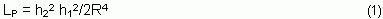where R is the horizontal distance between transmitter and receiver, h1 is the transmitter height, and h2 is the receiver height.

How did we get such a compact, easy-to-remember equation for path loss? The short answer is "ground bounce." In any location near the ground, the radio transmission takes a direct path and a ground bounce path from the transmitter to the receiver, as shown in Figure 1.The ground bounce contribution can be thought of as a reflection from a mirror. It is reflected with a 180° phase shift for conventional terrain, and travels a longer distance than does the direct contribution. The two contributions recombine at the receiver, where they would cancel completely if not for the path-length difference.

The direct and ground-bounce distances are given by Equations 2 and 3.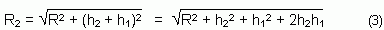For R, R1, R2 >> h1, h2, these expressions are approximated by Equation 4 and Equation 5.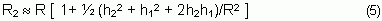The difference between the two distances is given by Equation 6.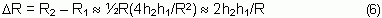The ground bounce is a simple example of multipath transmission: the transmitted radio wave bounces off of multiple surfaces, resulting in multiple signals with different amplitudes and delays arriving at the receiver.

In free space there is only one transmission path, and the signal power at the receiver is given by Equation 7 as:where PR is the received power, PT is the transmitted power, GT is the transmitter antenna gain, GR is the receive antenna gain, and λ is the wavelength.

Recall that when the ground is present, the transmitted power takes two paths: direct and ground bounce. There are many ways to model this transmission—most of them worthy of a graduate thesis. A reasonable and intuitive way to show the effect of the second path is to assume that half of the power goes into the direct path and half goes into the ground-bounce path. Consequently, there are two voltages with slightly different phases that subtract at the receive antenna (remember the 180° phase reversal of the reflection). Equation 8 shows the complex number representation for the combination of these two voltages.The two voltages, V1 and V2, are virtually the same in magnitude for most flat-ground conditions. We can consider V to be a "voltage" (in this case, volts/ohm1/2) that is equal to the square root of half the received power, or as in Equation 9:The power received is just the squared magnitude of the combined voltage in Equation 8.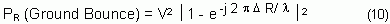Substituting V from Equation 9 into this, and combining the complex exponential terms into trigonometric functions reduces the equation for exact path loss to: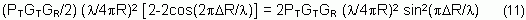If we substitute the approximation for ΔR from Equation 6 into Equation 11, and approximate sinx ≈ x, we get the following simplified expression:For small antennas with wide angular coverage the antenna gain is nearly unity. Expressing Equation 12 as a ratio of PR/PT and setting GT = GR = 1, leads to the approximation that is Equation 1.

Figures 2 and 3 show plots of these path loss equations at 315MHz and 434MHz for antennas with unity gain. Included are the free-space path loss of Equation 7, the exact path loss given by Equation 11, and the approximate path loss given by Equation 12. We can see that the exact path loss varies dramatically at close distances and depends on the signal frequency.

It is interesting to note from these two plots that, given the typical RKE geometry in Figure 1, the path loss at a 10-meter distance can be approximated by the free-space path loss. This is because the direct contribution and ground bounce contribution are separated in distance by about one quarter of a wavelength at 300MHz to 400MHz, resulting in a 90 degree phase difference. This means that the two contributions add neither constructively nor destructively.

At distances greater than 10 meters, however, the path loss varies as R-4, which means that the expression in Equation 1 is a very useful quick calculation for path loss at moderate to long distances from the vehicle. In fact, for equal transmission and reception height, h, the path loss in dB is simply: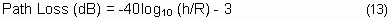This says that for a transmitter and receiver at 1-meter heights, the path loss at 1km is -123dB.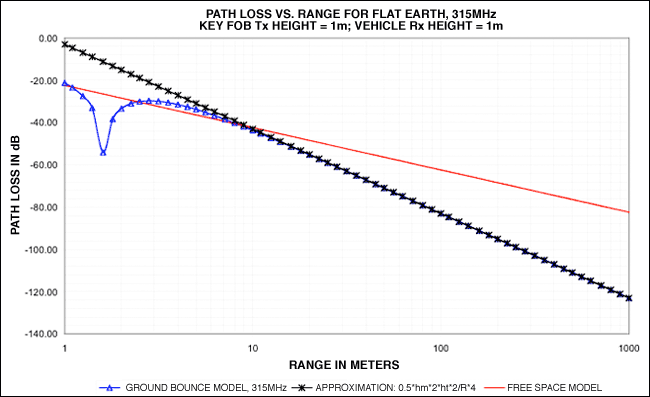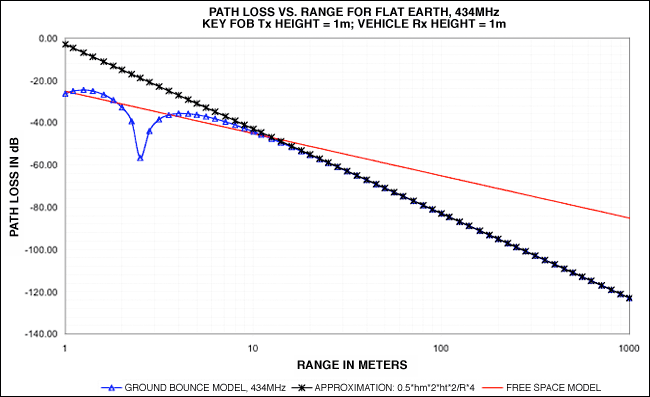### Tips for Using Path-Loss Calculations

The manner in which the power from the transmitter is split between the direct path and the ground path is not exact. This is why the expressions in Equations 12 and 13 sometimes have a factor of 2 variation, depending on the model. What is important, however, is that the expressions in this application note closely approximate the best range performance achievable and describe how path loss varies with height and distance.

The free-space loss model can be used for ranges under 10 meters from the vehicle, as long as you understand that wide variations can occur from the ground bounce reflection within that 10 meters. For ranges greater than 10 meters in obstruction-free environments, the R-4 approximation can be used.

The presence of any other scattering surfaces increases the variation in path loss at any given distance. Any obstacles (other cars in the parking lot, light poles, low buildings, etc.) create more bounce paths, diffract the radio waves, and in the case of concrete buildings, attenuate them. This means that the R-4 loss behavior, as bad as it seems compared to free-space loss, is actually very optimistic. In a realistic setting, a good guideline is to subtract 20dB from the "empty parking lot" loss in Equation 1 to allow for the instantaneous fading that comes from multiple surfaces. If the key fob is inside a building (for instance, in a remote start application), subtract 30dB to 40dB from the loss in Equation 1.

In the final analysis, the most reliable way to determine maximum range is through empirical testing. The approximations above are a reference point or "sanity check" from which to start your measurements.